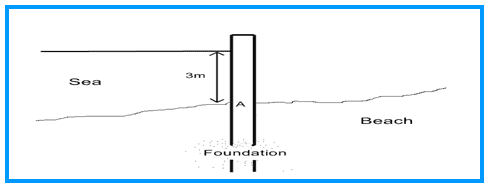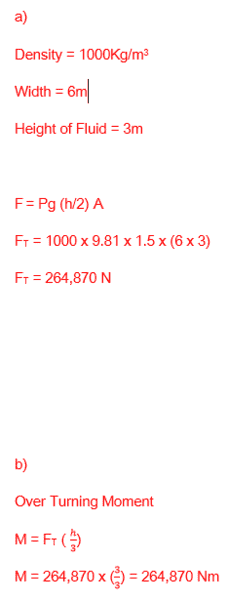# Hydrostatic Pressure

## Homework Statement

[/B]
Hello, am i on the right track with this problem. I am not sure if i have done the it correct. The part i am unsure on is b). Not sure if i have calculated it correct.

Part of a sea defence consists of a section of a concrete wall 4 metres high, and 6 metres wide. (See cross-section of the wall in the figure below).a) Calculate the resultant thrust on the section when the sea reaches a height of 3 metres relative to the base of the section.

b) Calculate the overturning moment experienced by the section about point A.

## The Attempt at a Solution#### Attachments

Last edited:

stockzahn
Homework Helper
Gold Member
You've used something like "empirical" formulas. It would be interesting to calculate the results by integrating the pressure forces over the height of the wall to check, if the formulas are correct. E.g. for a) starting from the top of the wall with ##x## as variable pointing downwards:

$$\int\limits_{x=0}^{x=3}Width\,\rho\,g\,x\,dx = Width\,\rho\,g \int\limits_{x=0}^{x=3}x\,dx = Width\,\rho\,g \bigg{|}_{x=0}^{x=3}\frac{x^2}{2}=6\cdot 1000\cdot 9.81 \cdot \left(\frac{3^2-0^2}{2}\right)=264870\;N$$

How about the calculation of the overturning momentum?

Thank you for replying. So is the formula i done incorrect? Because i see your formula got the same answer. For the overturning momentum i used m = Ft (h/3). Which was also 264,870 Nm. But i was getting confused with where the A is. And if i calculated it correct from the A. Thanks.

stockzahn
Homework Helper
Gold Member
That's why I proposed to do the calculation using another method to check your approach. I think it's a matter of some minutes, but it's be helpful in the future, especially if the pressure force distribution is not linear.

To obtain the overturning moment around point A you have to integrate the momenta of each pressure force, like

$$\int dM(x)$$.

Any idea how to express ##M(x)## to solve the integral?

Last edited:
now i understand why you done it that way. I don't really have an idea. Do you put 3 and 0 at the top and bottom of the intergral? And x would be 264870?

stockzahn
Homework Helper
Gold Member
I attached a small drawing (below the text). It shows the situation with the variable ##x##. At the position ##x## there is small piece of the wall (with the width ##w = 6\, m## and the height ##dx##). The pressure acts on this small area (##w\cdot dx##) and generates a small force ##dF##. This force ##dF## generates a small moment ##dM## turning around point A. But of course the moment is depending on ##x##. Therefore you have to find an expression for ##dM(x)##.

Start with that: What would be the turning moment of ##dF## around point A?

#### Attachments

stockzahn
Homework Helper
Gold Member
Next step would be to express ##dF## by means of the height-variable pressure, plug that all in into the integral and solve it. Sorry, have to leave. Good luck!

•Greg Bernhardt